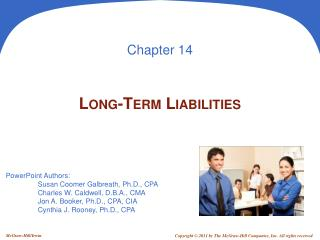DownloadDownload PresentationLong-Term Liabilities

# Long-Term Liabilities

Télécharger la présentation## Long-Term Liabilities

- - - - - - - - - - - - - - - - - - - - - - - - - - - E N D - - - - - - - - - - - - - - - - - - - - - - - - - - -
##### Presentation Transcript

1. Chapter 14 Long-Term Liabilities

2. Bond Financing A1 Advantages Disadvantages Bonds do not affect owner control. Bonds require payment of both periodic interest and par value at maturity. Interest on bonds is tax deductible. Bonds can decrease return on equity. Bonds can increase return on equity.

3. On Jan. 1, 2011, a company issued the following bonds: Par Value: \$800,000 Stated Interest Rate: 9% Interest Dates: 6/30 and 12/31 Maturity Date = Dec. 31, 2030 (20 years) Issuing Bonds at Par P1

4. Issuing Bonds at Par P1 On June 30, 2011, the issuer of the bond pays the first semiannual interest payment of \$36,000. \$800,000 × 9% × ½ year = \$36,000 This entry is made every six months until the bonds mature.

5. Issuing Bonds at Par P1 On December 31, 2030, the bonds mature and the issuer of the bond pays face value of \$800,000 to the bondholders.

6. Issuing Bonds at a Discount P2 Fila issues bonds with the following provisions: Par Value: \$100,000 Issue Price: 96.454% of par value Stated Interest Rate: 8% Market Interest Rate: 10% Interest Dates: 6/30 and 12/31 Bond Date: Dec. 31, 2011 Maturity Date: Dec. 31, 2013 (2 years) } Bond will sell at a discount.

7. Issuing Bonds at a Discount P2 On Dec. 31, 2011, Fila should record the bond issue. Contra-Liability Account

8. Issuing Bonds at a Discount P2 Maturity Value Carrying Value Amortizing a Bond DiscountUsing the straight-line method, the discount amortization will be \$887 (rounded) every six months. \$3,546 ÷ 4 periods = \$887 (rounded)

9. Amortizing a Bond Discount P2 Fila will make the following entry every six months to record the cash interest payment and the amortization of the discount. \$3,546 ÷ 4 periods = \$887 (rounded) \$100,000 × 8% × ½ = \$4,000

10. Issuing Bonds at a Premium P3 Adidas issues bonds with the following provisions: Par Value: \$100,000 Issue Price: 103.546% of par value Stated Interest Rate: 12% Market Interest Rate: 10% Interest Dates: 6/30 and 12/31 Bond Date: Dec. 31, 2011 Maturity Date: Dec. 31, 2013 (2 years) } Bond will sell at a premium.

11. Issuing Bonds at a Premium P3 On Dec. 31, 2011, Adidas will record the bond issue as: Adjunct-Liability Account

12. Issuing Bonds at a Premium P3 Maturity Value Carrying Value Amortizing a Bond PremiumUsing the straight-line method, the premium amortization will be \$887 (rounded) every six months. \$3,546 ÷ 4 periods = \$887 (rounded)

13. Amortizing a Bond Premium P3 Adidas will make the following entry every six months to record the cash interest payment and the amortization of the discount. \$3,546 ÷ 4 periods = \$887 (rounded) \$100,000 × 12% × ½ = \$6,000

14. Issuing Bonds at a Discount P2 Fila issues bonds with the following provisions: Par Value: \$100,000 Issue Price: ? Stated Interest Rate: 8% Market Interest Rate: 10% Interest Dates: 6/30 and 12/31 Bond Date: Dec. 31, 2011 Maturity Date: Dec. 31, 2013 (2 years)

15. Present Value of a Discount Bond P2 To calculate Present Value, we need relevant interest rate and number of periods. Semiannual rate = 5% (Market rate 10% ÷ 2) Semiannual periods = 4 (Bond life 2 years × 2) \$100,000 × 8% × ½ = \$4,000

16. Debt-to-Equity Ratio A3 Debt-to- Equity Ratio Total Liabilities Total Equity = This ratio helps investors determine the risk of investing in a company by dividing its total liabilities by total equity.

17. End of Chapter 14